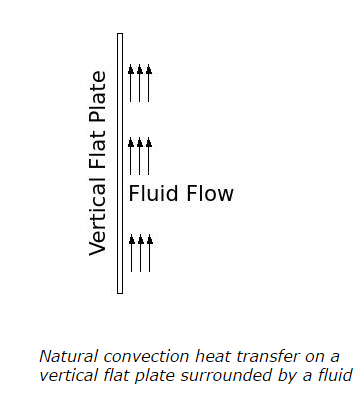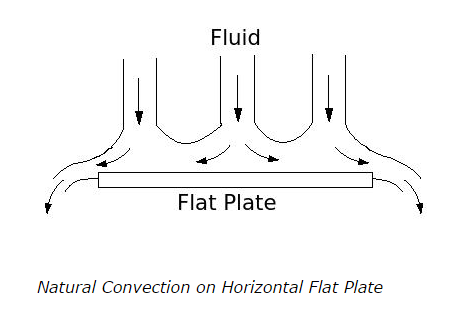# Natural Convection and Forced Convection - 1 - MCQs with Answers

## Natural Convection and Forced Convection – 1 – MCQs with Answers

1. Generally, natural convection occurs due to

a. change in velocity of a fluid
b. change in density of a fluid
c. change in molecular structure of a fluid
d. none of the above

ANSWER: b. change in density of a fluid

2. The buoyancy forces which give rise to the natural convection are called as

a. convection forces
b. fluid forces
c. body forces
d. none of the above

3. The intensity of mixing of fluid in natural convection is

a. more than the intensity of mixing of fluid in forced convection
b. less than the intensity of mixing of fluid in forced convection
c. equal to the intensity of mixing of fluid in forced convection
d. unpredictable

ANSWER: b. less than the intensity of mixing of fluid in forced convection

4. What is the relation between convection heat transfer coefficients of natural convection and forced convection?

a. convection heat transfer coefficient of natural convection is lower than the convection heat transfer coefficient of forced convection
b. convection heat transfer coefficient of natural convection is more than the convection heat transfer coefficient of forced convection
c. convection heat transfer coefficients in both natural and forced convection are the same for same system
d. unpredictable

ANSWER: a. convection heat transfer coefficient of natural convection is lower than the convection heat transfer coefficient of forced convection

5. Below figure shows a natural convection heat transfer on a vertical flat plate surrounded by a fluid.What is the relation between the upward velocity of the fluid and the distance from the bottom of the plate, when plate is hotter that fluid?

a. as the distance from the bottom of the plate increases, the upward velocity of the fluid near the plate surface decreases
b. as the distance from the bottom of the plate increases, the upward velocity of the fluid near the plate surface increases
c. the upward velocity of the fluid near the plate surface is same all over the plate
d. unpredictable

ANSWER: b. as the distance from the bottom of the plate increases, the upward velocity of the fluid near the plate surface increases

6. Assume a natural convection heat transfer on a vertical flat plate surrounded by a fluid. Where will be the fully developed turbulent layer of fluid established, if the plate is hotter than the fluid?

a. At the bottom of the plate
b. At the middle of the plate
c. At the top of the plate
d. Nowhere

ANSWER: c. At the top of the plate

7. Below figure shows a natural convection heat transfer on a horizontal flat plate and fluid is above it. Which condition satisfies the figure below?a. Plate temperature is lower than the fluid temperature
b. Plate temperature is higher than the fluid temperature
c. Plate temperature is equal to the fluid temperature
d. unpredictable